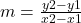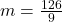## In 1990, the cost of tuition at a large Midwestern university was $95 per credit hour. In 1999, tuition had risen to$221 per credit hour. D

Question

In 1990, the cost of tuition at a large Midwestern university was $95 per credit hour. In 1999, tuition had risen to$221 per credit hour. Determine a linear function C(x) to represent the cost of tuition as a function of x, the number of years since 1990 C(x)= *answer here*

in progress 0
4 months 2021-09-04T16:59:28+00:00 1 Answers 5 views 0

The cost of tuition as a function of x, the number of years since 1990, is C(x)= 14*(x-1990) + 95

Step-by-step explanation:

A linear function is a polynomial function of the first degree that has the following form:

y= m*x + b

where

• m is the slope of the function
• n is the ordinate (at the origin) of the function

So, in this case: C(x)= m*( x-1990) + b where x is the number of years since 1990.

Given the coordinates of two points, it is possible to determine the slope m of the line from them using the following formula:In this case, you know that in 1990, the cost of tuition at a large Midwestern university was $95 per credit hour. And in 1999, tuition had risen to$221 per credit hour. So:

• x1= 1990
• y1= 95
• x2= 1999
• y2= 221

So the value of m is:m= 14

So C(x)= 14*( x-1990) + b. In 1999, tuition had risen to \$221 per credit hour. Replacing:

221= 14*(1999 – 1990) + b

221= 14*9 +b

221= 126 + b

221 – 126= b

95= b

Finally, the cost of tuition as a function of x, the number of years since 1990, is C(x)= 14*(x-1990) + 95